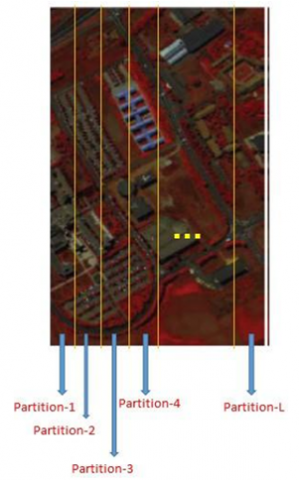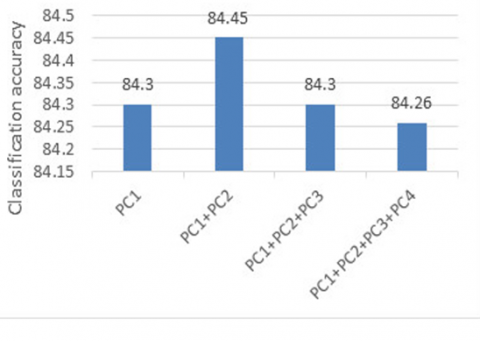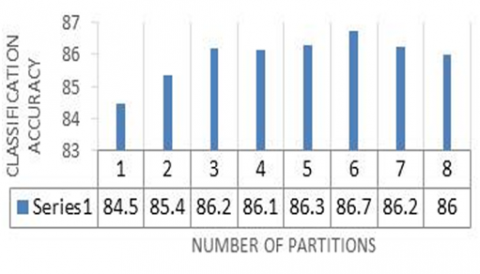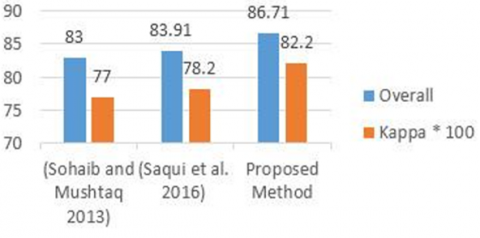# An Automatic Method for Unsupervised Feature Selection of Hyperspectral Images Based on Fuzzy Clustering of Bands

An Automatic Method for Unsupervised Feature Selection of Hyperspectral Images Based on Fuzzy Clustering of Bands
Faculty of Geodesy and Geomatics, K. N. Toosi University of Technology, Tehran 19967-15433, Iran

Corresponding Author Email:
b_asghari@email.kntu.ac.ir
Page:
319-324
|
DOI:
https://doi.org/10.18280/ts.370218
Received:
27 December 2019
|
Accepted:
16 February 2020
|
Published:
30 April 2020
| Citation

OPEN ACCESS

Abstract:

Hyperspectral sensors collect spectral data in numerous adjacent spectral bands which are usually redundant and cause some challenges such as Hughes phenomenon. In this study, an automatic unsupervised method is presented for feature selection from hyperspectral images. To do so, a new statistical feature space is introduced in which each band is regarded as a sample point. This feature space is originated from the statistical attributes of image bands while these attributes are extracted from different partitions of the entire image. A fuzzy clustering of bands, performed in the PCA transformed space of previously mentioned statistical feature space, leads to band clusters with similar characteristics. The proposed band selection technique chooses a representative band in each cluster and removes the other redundant ones. The proposed method is investigated in terms of the classification accuracy of the Pavia University hyperspectral image. Obtained results, which are compared to two recent states of art methods, prove its efficiency.

Keywords:

hyperspectral classification, band selection; statistical attributes, fuzzy c-means clustering, virtual dimensionality, principal component analysis

1. Introduction

Hyperspectral sensors collect data simultaneously in hundreds of narrow and adjacent spectral bands over the wavelengths from the near-ultraviolet through the thermal infrared and form a data cube that its third dimension specified by wavelengths. These sensors have great ability in the accurate reconstruction of the spectral signature curve which is a powerful tool for the identification of ground materials .

Redundant adjacent bands in a hyperspectral image cause storage and transmission challenges, increasing computational complexity and commonly, can decrease the performance of the classifiers . Dimensionality reduction is a strategy to deal with the mentioned challenges. For hyperspectral remote sensing, dimensionality reduction references to compress the number of the bands without the decrease of the useful information . Generally, dimensionality reduction methods can be categorized into two main groups; feature extraction and feature selection. The main goal of feature extraction is to find a transformation that maps data to the new low dimensional space which preserves essential discriminative information  but Feature selection methods attempt to select a group of bands with more information and less correlation.

Both dimensionality reduction categories again can categorize into two main subgroups: unsupervised and supervised whether it uses the labeled samples or not. In the subgroup of unsupervised feature extraction, there are well-known methods such as Principal component analysis (PCA), independent components analysis (ICA), and minimum noise fraction (MNF) which ranks the extracted features based on some criteria .

There are numerous improved versions of these traditional methods in literature such as; segmented principal components analysis , non-linear PCA , Superpixel-based PCA (SuperPCA) , and segmented minimum noise fraction  for classification of hyperspectral images. In addition to these traditional approaches, there are some advanced nonlinear manifold-based methods such as; locality preserving projection (LPP)  and its new two stage version (TwoSP)  for classification of hyperspectral images. In the recent years some pixel-based methods such wavelet decomposition , rational curve fitting  and fractal dimension of spectral response curve  are proposed which considered the spectral curves of each pixel for extracting the new features. In the subgroup of supervised feature extraction, there are methods such as decision boundaries (DBFE) , nonparametric weighted feature extraction (NWFE) , linear discriminant analysis (LDA) , spectral segmentation and integration (SSI) based on PSO optimization .

Feature selection methods can be categorized into six subgroups as below :

(1) Ranking based methods: in these methods the importance of each feature is computed and bands are sorted based on their importance and the desired number of features is selected from top-ranked ones. Information divergence  and mutual information  are the most important unsupervised and supervised methods of this subgroup.

(2) Searching-based methods: In these methods band selection problem considered as an optimization problem. Integration genetic and PSO  and PSO with dynamic sub-swarms  are the recent methods of this subgroup.

(3) Sparsity-based methods: In these methods band selection problem considered as a sparsity constraint optimizati

2. Methodology

As mentioned earlier, MAD and kurtosis measures are used as statistical measures . It seems that using the more statistical measures can enhanced their method. As an example, consider two vectors as below:

A=[1.13, 2.87, -0.38, -0.31, -0.11, 1.91, 1.17, -0.36, 1.71, 0.29]

B=[1.87, 2.55, -0.074, 0.879, 0.058, 1.63, 0.22, 0.012, 1.46, 1.066].

Some statistical measures of these two vectors are as Table 1.

It can be understood that the largest difference between the two vectors is in iqr measure. As the results in this study, some additional statistical measures (Table 2) are considered in comparison to  to better describe the hyperspectral bands.

Table 1. Some statistical measures of vectors A and B

 A B Mean 0.79 0.96 MAD 0.96 0.75 Kurtosis 1.97 1.86 Inter quartile range (iqr) 2.02 1.57

Table 2. Statistical attributes of bands

 Statistical attributes Formula Mean absolute deviation (MAD) $\mathrm{SM} 1_{\mathrm{b}}=\frac{1}{M \cdot N} \sum_{i=1}^{M \cdot N}\left|B_{i}-\operatorname{mean}(b)\right|$ Standard deviation (STD) $\mathrm{SM} 2_{\mathrm{b}}=\sqrt{\frac{1}{M \cdot N} \sum_{i=1}^{M \cdot N}\left(B_{i}-\operatorname{mean}(b)\right)^{2}}$ Variance (Var) $\mathrm{SM} 2_{\mathrm{b}}=\frac{1}{M \cdot N} \sum_{i=1}^{M \cdot N}\left(B_{i}-\operatorname{mean}(b)\right)^{2}$ Level-3 Moment $\mathrm{SM} 4_{\mathrm{b}}=\mathrm{E}\left(\left(B_{i}-\operatorname{mean}(b)\right)^{3}\right)$ Mean $\mathrm{SM} 5_{\mathrm{b}}=\frac{\sum_{i=1}^{M \cdot N} B_{i}}{M \cdot N}$ Median For B1=min, B2,…, BM.N=max If M.N is odd $\mathrm{SM} 6_{\mathrm{b}}=B_{\frac{(M \cdot N+1)}{2}}$ If M.N is even $\mathrm{SM} 6_{\mathrm{b}}=\frac{1}{2}\left(B_{\frac{(M \cdot N)}{2}}+B_{1+\frac{(M \cdot N)}{2}}\right)$ Kurtosis $\mathrm{SM} 7_{\mathrm{b}}=\frac{\frac{1}{M \cdot N} \sum_{i=1}^{M . N}\left(B_{i}-(\operatorname{mean}(b))^{4}\right.}{\left(\frac{1}{M \cdot N} \sum_{i=1}^{M . N}\left(B_{i}-(\operatorname{mean}(b))^{2}\right)^{2}\right.}$ Skewness $\mathrm{SM} 8 \mathrm{b}=\frac{\frac{1}{M \cdot N} \sum_{i=1}^{M \cdot N}\left(B_{i}-(\operatorname{mean}(b))^{3}\right.}{\sqrt{\left(\frac{1}{M \cdot N} \sum_{i=1}^{M . N}\left(B_{i}-(\operatorname{mean}(b))^{2}\right)^{3}\right.}}$ Inter quartile range (irq) SM9b=Q3-Q1

In Table 2, Bi is value of $i^{t h}$ pixel, M and N show image dimensions, $\operatorname{mean}(b)$ is mean of all pixel values in band b and $Q 3, Q 1$ are third and first quartile. Figure 1 shows the flowchart of the proposed method. The details are presented below:

## 1.pngFigure 1. Flowchart of proposed method

Noisy bands (such as water vapor bands) have no useful information and thus the proposed method begins by removing them manually via visual inspection. The remained bands are then introduced to Harsanyi, Farrand, and Chang (HFC), well-known virtual dimensionality (VD) estimation algorithm , to estimate the number of distinct signatures.

Image-space is spatially partitioned to equal size segments (Figure 2). This is due to the elongation of the image object illustrated along the width of the image (such as road and buildings). An attribute feature space is then formed by extracting some statistical attributes from each image segment and for each hyperspectral band. It can help our method catches the local characteristics of each band in comparison to other previous methods.

## 2.pngFigure 2. Image partitioning

These nine features, if extracted from L image partition, lead to 9×L attributes for each band which means that bands can be regarded as points in that 9×L dimension space. In other words, band b can be described by a vector in the form of Eq. (1):

$\boldsymbol{b}=[S M 11, \ldots, S M 91, \ldots, S M 1 L, \ldots, S M 1 L]^{T}$     (1)

where, $S M 11, \ldots, S M 91$ represent the nine statistical attributes of Table 2 and L is the number of image partitions. The components of Eq. (1) are the statistical attributes of the bands. In the next stage, a PCA was applied to band attributes space for extracting the most useful attributes of each band. PCA is the linear transformation that aims to find transformation matrix that transform the original features space b to the new uncorrelated feature space y as below:

$y_{i}=W^{T} b_{i}$    (2)

Each hyperspectral band can be represented by a 9×L directional vector as b=[SM1, SM2,…,SM9×L]. If hyperspectral image contains the M bands the covariance matrix of x is computed as :

$\operatorname{cov}(b)=E\left\{\left(b-E(b)\left(b \cdot-E(b)^{T}\right)\right\}\right.$     (3)

where, E is expectation operator and T is the transpose. The covariance matrix can be represented in eigenvalue decomposition as below:

$\operatorname{cov}(x)=W D W^{T}$     (4)

where, D is the diagonal matrix which consists of eigenvalues of cov(x) and W is the orthogonal matrix of eigenvectors of cov(x). Commonly, eigenvectors correspond to the k largest eigenvalues are used in (2) as the transformation matrix .

As the same motivation which is proposed by Saqui at al. , Fuzzy c-means clustering was applied to cluster the bands in the principal components space for VD number of clusters. Fuzzy c-means clustering aims to minimize of the following objective function :

$J_{m}=\sum_{i=1}^{M} \sum_{i=1}^{V D} u_{i j}^{m}\left\|b_{i}-c_{j}\right\|^{2} \quad 1 \leq m \leq \infty$     (5)

where, uij is the degree of membership of bi in the cluster j, cj center of the jth cluster. An iterative method is carried out to solve the above optimization problem with update of membership and cluster center as below :

$u_{i, j}=\frac{1}{\sum_{k=1}^{V D}\left(\frac{\left\|b_{i}-c_{j}\right\|}{\left\|b_{i}-c_{k}\right\|}\right)^{\frac{2}{m-1}}}$     (6)

$c_{j}=\frac{\sum_{i=1}^{M} u_{i}^{m} \cdot b_{i}}{\sum_{i=1}^{M} u_{i j}^{m}}$     (7)

The iterative procedure will stop when the changes in the value of ui,j is small. It is assumed that the bands with similar information content would appear to have high membership values to common clusters. Accordingly, a band with a maximum degree of membership was selected as the representative band of each cluster and the other bands were removed as they were thought to be redundant.

3. Data Set

The data set of this study is acquired by the ROSIS-3 sensor from Pavia University from in northern Italy on 8 July 2002 and has a size of 610 × 340 pixels with 1.3m spatial resolution. Each image pixel contains 115 reflectance values from 0.43 to 0.86 micrometers of the spectrum. 12 noisy bands were removed and the remaining 103 bands were used in this study. Pavia University's three-channel color composite and ground-truth map are shown in Figure 3. In addition, Table 3 shown the total numbers of samples in each class based on ground truth.

Table 3. Ground truth samples distribution

 Class No. of sample in ground truth asphalt 6631 Meadows 18649 Gravel 2099 Trees 3064 Painted metal sheets 1345 Bare Soil 5029 Bitumen 1330 Self-Blocking Bricks 3682 Shadows 947 Total 42776

## 3.png(a) Color composite image (b) Ground truth map

Figure 3. Pavia University

4. Experimental Results

The VD of Pavia University data set was estimated by HFC based on 10−5 as a false alarm which found to be 13 dimensions. This VD number determines the number of clusters in the latter fuzzy c-means clustering. Due to the randomness of the fuzzy c-mean clustering initialization, clustering was performed ten times and the one with the lowest clustering objective function  was chosen.  The proposed band selection method was evaluated in terms of classification accuracy based on overall accuracy (OA) and kappa coefficient (Kappa) measures as below:

$\mathrm{O} \mathrm{A}=\frac{a}{A}$    (8)

$k a p p a=\frac{A \sum_{i=1}^{r} X_{i i}-\sum_{i=1}^{r} X_{i+} X_{+i}}{A^{2}-\sum_{i=1}^{r} X_{i+} X_{+i}}$      (9)

where, in 8, A and a are the total number of test and correctly classified samples, respectively. In (9), Xii is the total number of samples belonging to the class i that have also been classified as class i (diagonal elements of the confusion matrix). Xi+ and X+i are the sum of all columns and all rows of error matrix for class i. Also, r is the total number of classes in ground truth.

For the sake of the classification, selected bands (features) are classified based on K-nearest neighbor (K-NN) classification. K-nearest neighbor (K-NN) algorithm is the non-parametric supervised simple classification method that has the appropriate results in the classification of hyperspectral images.  In this method the label of each test sample is determined based on the K-nearest labeled sample. Commonly Euclidean metric (EM) is used to measure the distance between each test (x) and labeled (y) sample as below :

$E M(x, y)=\sqrt{\sum_{i=1}^{n}\left|x_{i}-y_{i}\right|^{2}}$     (10)

where, n is the number of the features. Training samples were randomly selected as the 10% of ground truth samples and the remaining ones were applied to evaluate the classification results.

4.1 Effects of PCs

In the first experiment, image partitioning was not performed and only nine statistical attributes were calculated for each band of hyperspectral image. PCA was applied to these band statistical attributes and led to new uncorrelated principal components (PCs). Different numbers of PCs, from 1 to 4, were used in the proposed band selection to examine the effects of this parameter. Reduced data of each case was evaluated using overall classification accuracy (Figure 4).

## 4.pngFigure 4. Effect of different number of PCs

4.2 Effects of image partitioning

The second experiment aims to evaluate the effects of image partitioning on the performance of the proposed method. To do so, different numbers of image partitions (i.e. 1 to 8) were evaluated. The obtained results are presented in Figure 5.

Figure 5 suggests that image partitioning has significant effects on the performance of the proposed method. This means that statistical attributes associated to each band have local nature. The optimum number of image partitions is 6 in our case. This type of partitioning is used due to its simplicity and very fast nature. But more precisely, this parameter, as well as the proper method of image partitioning, needs to be the subject of more research in future.  According to Figure 5, even with two image partitions classification accuracy of selected bands exceed the minimum mapping accuracy of 85% which is required for most resource management applications .

## 5.pngFigure 5. Effect of image partisioning

4.3 Comparison to other methods

The proposed method was compared to the studies  and  which are the most recent unsupervised band selection techniques with a similar concept to the proposed method. Figure 6 shows the obtained results which indicate the superiority of the proposed method. Both of the two opponent methods, as well as the proposed method, introduce their selected bands based on band clustering. The superiority of the proposed method can be traced to two factors:

(1) The rich original statistical attributes which were assigned to each band (Table 2).

(2) The strategy of image partitioning which describes the bands more efficiently.

## 6.pngFigure 6. Results of different methods

In comparison to classification with original spectral bands (overall= 86.16), only our proposed method is /superior. This indicates that our method can efficiently reduce the dimension of data to about 0.14 along with the preservation of important original data. Besides, although the proposed method is feature selection, it is still more precise than the PCA feature extraction method (13 first PCs) with an overall accuracy of 86.15%.

5. Conclusion

In this paper, an automatic unsupervised band selection technique was proposed for the sake of dimensionality reduction of hyperspectral images. The underlying concept of the proposed method is assigning some statistical attributes to each band and then clustering them accordingly. A representative band in each cluster was kept and the remaining ones were assumed to be redundant. To examine the performance of the proposed method, the resultant bands were used in K-NN classification and the results were evaluated in terms of overall accuracy and kappa coefficients for the test samples. The accuracy results of the proposed method are higher than the other two recent methods of this field and the PCA feature extraction method when the same set of training and test samples is chosen which proved the efficiency of the proposed method.

The band selection method of this paper applied an image partition that has a significant impact on its functionality. Determination of the proper number and strategy of this partitioning is proposed for further study.

References

 Wei, Y., Zhu, X., Li, C., Guo, X., Yu, X., Chang, C., Sun, H. (2017). Applications of hyperspectral remote sensing in ground object identification and classification. Advances in Remote Sensing, 6(3): 201-211. https://doi.org/10.4236/ars.2017.63015

 Hughes, G. (1968). On the mean accuracy of statistical pattern recognizers. IEEE Transactions on Information Theory, 14(1): 55-63. https://doi.org/10.1109/TIT.1968.1054102

 Su, H., Sheng, Y., Du, P. (2008). A new band selection algorithm for hyperspectral data based on fractal dimension. The International Archives of the Photogrammetry, Remote Sensing and Spatial Information Sciences, 37(B7): 279-284.

 Hosseini, S.A., Ghassemian, H. (2016). Rational function approximation for feature reduction in hyperspectral data. Remote Sensing Letters, 7(2): 101-110. https://doi.org/10.1080/2150704X.2015.1101180

 Chang, C.I. (2013). Hyperspectral data processing: algorithm design and analysis. John Wiley & Sons.

 Jia, X., Richards, J.A. (1999). Segmented principal components transformation for efficient hyperspectral remote-sensing image display and classification. IEEE transactions on Geoscience and Remote Sensing, 37(1): 538-542. https://doi.org/ 10.1109/36.739109

 Licciardi, G., Chanussot, J. (2018). Spectral transformation based on nonlinear principal component analysis for dimensionality reduction of hyperspectral images. European Journal of Remote Sensing, 51(1): 375-390. https://doi.org/10.1080/22797254.2018.1441670

 Jiang, J., Ma, J., Chen, C., Wang, Z., Cai, Z., Wang, L. (2018). SuperPCA: A superpixelwise PCA approach for unsupervised feature extraction of hyperspectral imagery. IEEE Transactions on Geoscience and Remote Sensing, 56(8): 4581-4593. https://doi.org/10.1109/TGRS.2018.2828029

 Guo, L.X., Xie, W.X., Pei, J.H. (2015). Segmented minimum noise fraction transformation for efficient feature extraction of hyperspectral images. Pattern Recognition, 48(10): 3216-3226. https://doi.org/10.1016/j.patcog.2015.04.013

 He, X., Niyogi, P. (2004). Locality preserving projections. In Advances in Neural Information Processing Systems, pp. 153-160.

 Li, X., Zhang, L., You, J. (2018). Hyperspectral image classification based on two-stage subspace projection. Remote Sensing, 10(10): 1565. https://doi.org/10.3390/rs10101565

 Prabhu, N., Arora, M.K., Balasubramanian, R. (2016). Wavelet based feature extraction techniques of hyperspectral data. Journal of the Indian Society of Remote Sensing, 44(3): 373-384. https://doi.org/10.1007/s12524-015-0506-9

 Ghosh, J.K., Somvanshi, A. (2008). Fractal-based dimensionality reduction of hyperspectral images. Journal of the Indian Society of Remote Sensing, 36(3): 235-241. https://doi.org/10.1007/s12524-0080024-0

 Lee, C., Landgrebe, D.A. (1993). Feature extraction based on decision boundaries. IEEE Transactions on Pattern Analysis and Machine Intelligence, 15(4): 388-400. https://doi.org/ 10.1109/34.206958

 Kuo, B.C., Landgrebe, D.A. (2004). Nonparametric weighted feature extraction for classification. IEEE Transactions on Geoscience and Remote Sensing, 42(5): 1096-1105. https://doi.org/ 10.1109/TGRS.2004.825578

 David, L. (2002). Hyperspectral image data analysis as a high dimensional signal processing problem. IEEE Signal Processing Mag, 19(1): 17-28.

 Moghaddam, S.H.A., Mokhtarzade, M., Beirami, B.A. (2020). A feature extraction method based on spectral segmentation and integration of hyperspectral images. International Journal of Applied Earth Observation and Geoinformation, 89: 102097. https://doi.org/10.1016/j.jag.2020.102097

 Sun, W., Du, Q. (2019). Hyperspectral band selection: A review. IEEE Geoscience and Remote Sensing Magazine, 7(2): 118-139. https://doi.org/10.1109/MGRS.2019.2911100

 Chang, C.I., Liu, K.H. (2013). Progressive band selection of spectral unmixing for hyperspectral imagery. IEEE Transactions on Geoscience and Remote Sensing, 52(4): 2002-2017. https://doi.org/10.1109/TGRS.2013.2257604

 Guo, B., Gunn, S.R., Damper, R.I., Nelson, J.D. (2006). Band selection for hyperspectral image classification using mutual information. IEEE Geoscience and Remote Sensing Letters, 3(4): 522-526. https://doi.org/10.1109/LGRS.2006.878240

 Ghamisi, P., Benediktsson, J.A. (2014). Feature selection of hyperspectral data by considering the integration of genetic algorithms and particle swarm optimization. In Image and Signal Processing for Remote Sensing XX, 9244: 92440J. https://doi.org/10.1117/12.2065472

 Xu, M., Shi, J., Chen, W., Shen, J., Gao, H., Zhao, J., (2018). A band selection method for hyperspectral image based on particle swarm optimization algorithm with dynamic sub-swarms. Journal of Signal Processing Systems, 90(8-9): 1269-1279. https://doi.org/10.1007/s11265-018-1348-9

 Li, J.M., Qian, Y.T. (2011). Clustering-based hyperspectral band selection using sparse nonnegative matrix factorization. Journal of Zhejiang University Science C, 12(7): 542-549. https://doi.org/10.1631/jzus.C1000304

 Du, Q., Bioucas-Dias, J.M., Plaza, A. (2012). Hyperspectral band selection using a collaborative sparse model. In IEEE International Geoscience and Remote Sensing Symposium, pp. 3054-3057. https://doi.org/10.1109/IGARSS.2012.6350781

 Li, H., Wang, Y., Duan, J., Xiang, S., Pan, C. (2013). Group sparsity based semi-supervised band selection for hyperspectral images. In IEEE International Conference on Image Processing, pp. 3225-3229. https://doi.org/10.1109/ICIP.2013.6738664

 Zhang, R., Ma, J., Chen, X., Tong, Q. (2009). Feature selection for hyperspectral data based on modified recursive support vector machines. In IEEE International Geoscience and Remote Sensing Symposium, 2: II-847. https://doi.org/10.1109/IGARSS.2009.5418228

 Mojaradi, B., Emami, H., Varshosaz, M., Jamali, S. (2008). A novel band selection method for hyperspectral data analysis. The International Archives of the Photogrammetry, Remote Sensing and Spatial Information Sciences, Beijing, China, pp. 447-454.

 Beirami, B.A., Mokhtarzade, M. (2019). Introducing an unsupervised method for feature extraction of hyperspectral images using bands clustering in the prototype space. Journal of Geomatics Science and Technology, 9(2): 195-207.

 Datta, A., Ghosh, S., Ghosh, A. (2015). Combination of clustering and ranking techniques for unsupervised band selection of hyperspectral images. IEEE Journal of Selected Topics in Applied Earth Observations and Remote Sensing, 8(6): 2814-2823. https://doi.org/10.1109/JSTARS.2015.2428276

 MartÍnez-UsÓMartinez-Uso, A., Pla, F., Sotoca, J. M., García-Sevilla, P. (2007). Clustering-based hyperspectral band selection using information measures. IEEE Transactions on Geoscience and Remote Sensing, 45(12): 4158-4171. https://doi.org/10.1109/TGRS.2007.904951

 Sohaib, M., Mushtaq, Q. (2013). Dimensional reduction of hyperspectral image data using band clustering and selection based on statistical characteristics of band images. International Journal of Computer and Communication Engineering, 2(2): 101. https://doi.org/10.7763/IJCCE.2013.V2.148

 Saqui, D., Saito, J.H., Campos, J.R., Jorge, L.A.D.C. (2016). Approach based on fuzzy c-means for band selection in hyperspectral images. International Journal of Computer and Information Engineering, 10(5): 889-895. https://doi.org/10.5281/zenodo.1124265

 Harsanyi, J.C., Farrand, W.H., Chang, C.I. (1993). Determining the number and identity of spectral endmembers: An integrated approach using Neyman-Pearson eigen-thresholding and iterative constrained RMS error minimization. In Proceedings of the Thematic Conference on Geologic Remote Sensing, pp. 395-395.

 Rodarmel, C., Shan, J. (2002). Principal component analysis for hyperspectral image classification. Surveying and Land Information Science, 62(2): 115-122.

 Bezdek, J. C., Ehrlich, R., Full, W. (1984). FCM: The fuzzy c-means clustering algorithm. Computers & Geosciences, 10(2-3): 191-203. https://doi.org/10.1016/0098-3004 (84)90020-7

 Prasatha, V.S., Alfeilate, H.A.A., Hassanate, A.B., Lasassmehe, O., Tarawnehf, A.S., Alhasanatg, M.B., Salmane, H.S.E. (2017). Effects of distance measure choice on KNN classifier performance-a review. arXiv preprint arXiv:1708.04321.

 Townshend, J.R., Hardy, J.R., Justice, C.O., Williams, D.F., Mitchell, C.W., Cook, A., Hancock, P. (1981). Terrain Analysis and Remote Sensing. London: George Allen & Unwin.